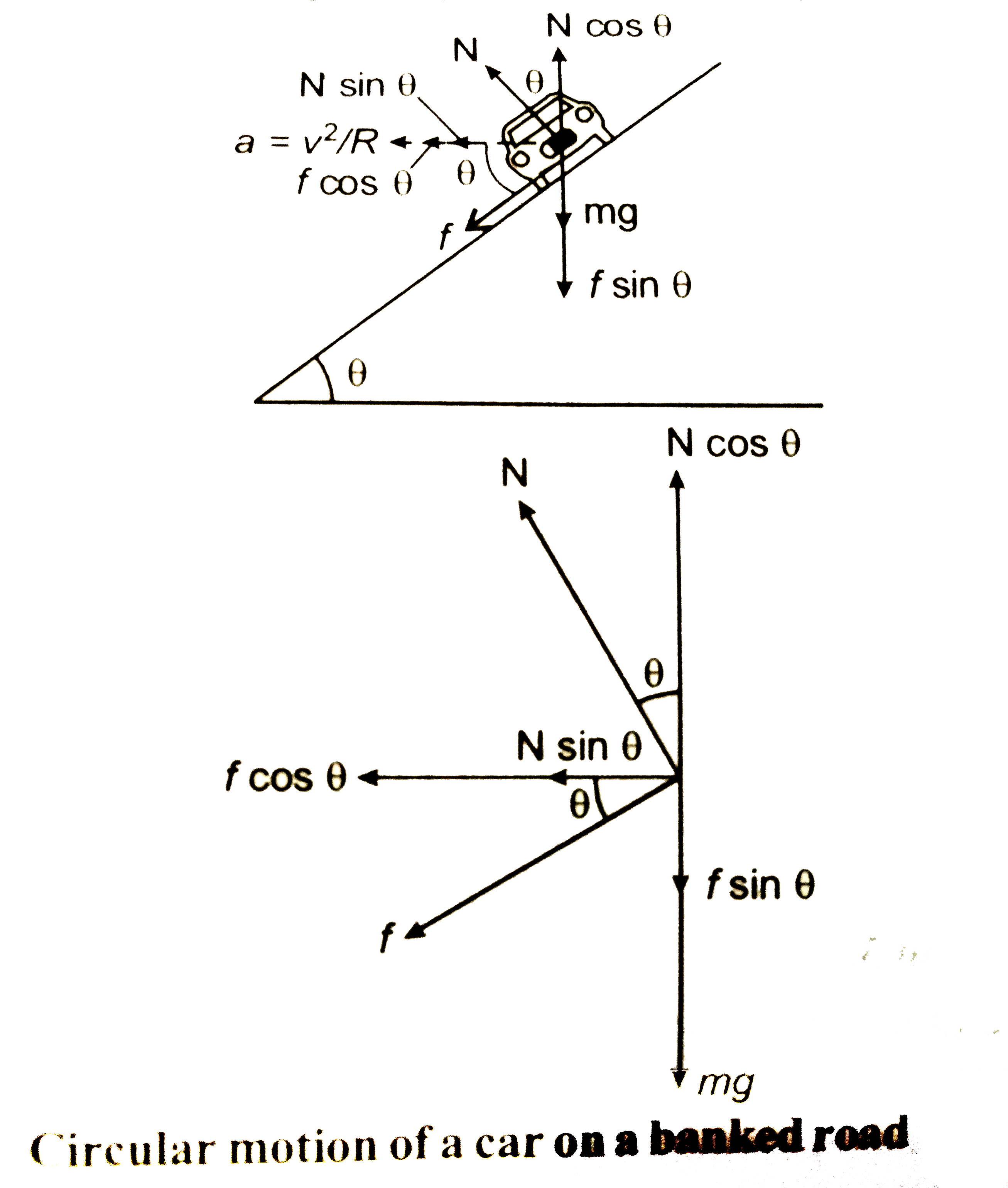# Draw neat, labelled diagram showing different forces acting on a vehicle moving along a banked road.

193 views
in Physics
closed
Draw neat, labelled diagram showing different forces acting on a vehicle moving along a banked road.

by (91.7k points)
selectedCircular motion of a car on a banked road
Where,
mg= Weight of vehicle
N= Normal reaction exerted on vehicle
f = Frictional force
theta = Angle of banking
a=(v^(2))/(R) = Centripetal acceleration.
fcostheta = Horizontal component of frictional force
fsintheta= Vertical component of frictional force
Ncostheta Vertical component of normal reaction.
Nsintheta = Horizontal component of normal reaction.

+1 vote